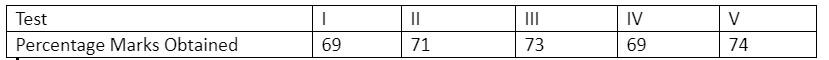Courses
Courses for Kids
Free study material
Free LIVE classes
MoreLIVE
Join Vedantu’s FREE Mastercalss

# The percentage of the marks obtained by a student in the monthly test are given below:Find the probability that a student gets more than 70% marks.Verified
According to the question, the probability that a student gets more than 70% marks $= \dfrac{{{\text{No}}{\text{. of test in which the student scored more than 70% marks}}}}{{{\text{total number of tests}}}}$ which is equal to $\dfrac{3}{5} = 0.6$.